# GMAT Math : DSQ: Calculating whether acute / obtuse triangles are congruent

## Example Questions

### Example Question #19 : Acute / Obtuse Triangles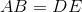and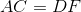Is it true that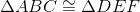?

1)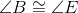2)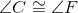Statement 1 ALONE is sufficient to answer the question, but Statement 2 ALONE is not sufficient.

EITHER statement ALONE is sufficient to answer the question.

Statement 2 ALONE is sufficient to answer the question, but Statement 1 ALONE is not sufficient.

BOTH statements TOGETHER are insufficient to answer the question.

BOTH statements TOGETHER are sufficient to answer the question, but NEITHER statement ALONE is sufficient to answer the question.

BOTH statements TOGETHER are sufficient to answer the question, but NEITHER statement ALONE is sufficient to answer the question.

Explanation:

If only one of the statements is known to be true, the only congruent pairs that are known between the triangles comprise two sides and a non-included angle; this information cannot prove congruence between the triangles. If both are known to be true, however, they, along with either of the given side congruences, set up the conditions for the Angle-Angle-Side Theorem, and the triangles can be proved congruent.

The answer is that both statements together are sufficient to answer the question, but not either alone.

### Example Question #20 : Acute / Obtuse Triangles

You are given two triangles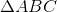and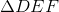; with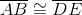and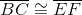. Which side is longer,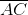or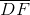?

Statement 1: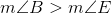Statement 2: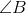and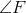are both right angles.

EITHER statement ALONE is sufficient to answer the question.

BOTH statements TOGETHER are sufficient to answer the question, but NEITHER statement ALONE is sufficient to answer the question.

BOTH statements TOGETHER are insufficient to answer the question.

Statement 2 ALONE is sufficient to answer the question, but Statement 1 ALONE is not sufficient to answer the question.

Statement 1 ALONE is sufficient to answer the question, but Statement 2 ALONE is not sufficient to answer the question.

EITHER statement ALONE is sufficient to answer the question.

Explanation:

We are given two triangles with two side congruences between them. If we compare their included angles (the angles that they form), the angle that is of greater measure will have the longer side opposite it. This is known as the Hinge Theorem.

The first statement says explicitly that the first included angle,, has greater measure than the second,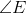, so the side opposite, has greater measure than.

The second statement is not so explicit. But ifis a right angle,must be acute, and ifis right, then, which again proves that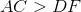.

The answer is that either statement alone is sufficient to answer the question.

Tired of practice problems?

Try live online GMAT prep today.